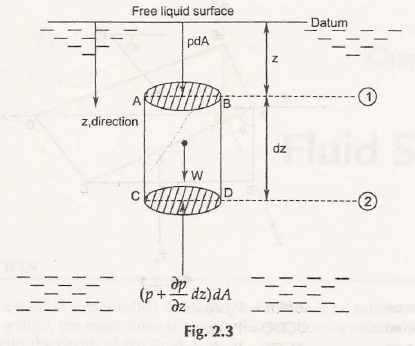Consider a cylinder is at rest. The fluid element of cylinder with the action of forces is shown in Fig.2.3Let pdA be the force on section AB at a distance z from the datum.

dz be the height of cylinder

(p + ∂p/∂zdz)dA be the force on section CD whose distance from datum is (z + dz)

W be the weight of cylinder

Then,

Forces can be written as (in z-direction),

∑▒Fz = 0

pdA + W – (p + ∂p/∂zdz)dA = 0

or,

∂p/∂zdzdA = W

Or,

w = ∂p/∂z

ρ . g = dp/dz            (ρ = Mass Density)

This suggests that rate of depth dp/dzis equal to the weight density of fluid.

The above equation can also written as-

p2 – p1= ρ. g (z2 – z1)

or,

p =  . g .z

Links of Next Mechanical Engineering Topics:-### Customer Reviews

My Homework Help
Rated 5.0 out of 5 based on 510 customer reviews at